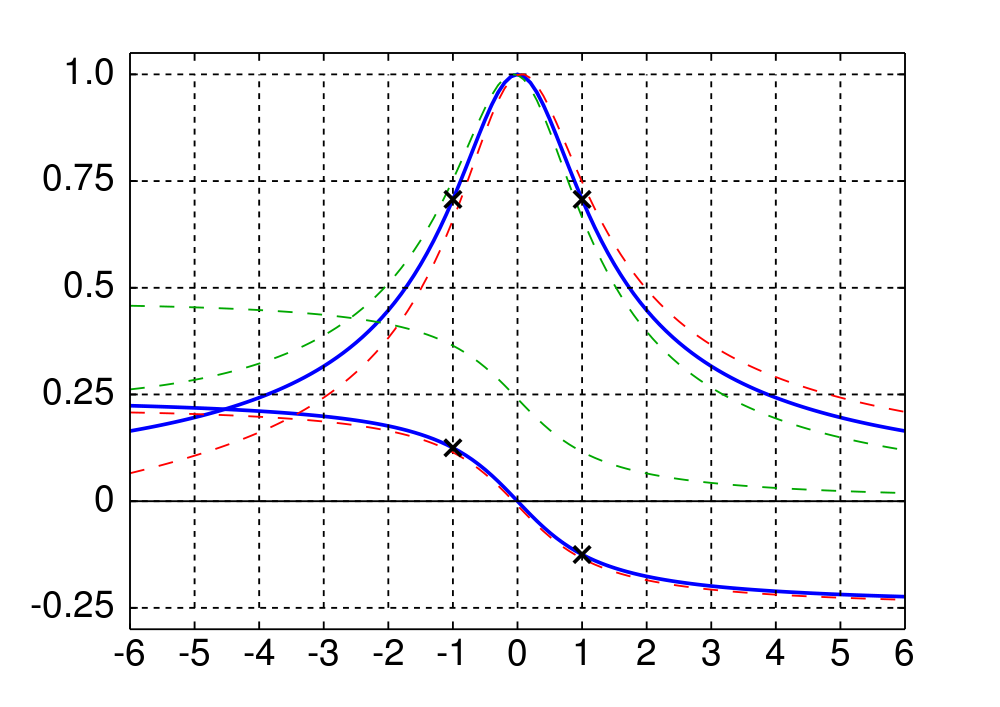## Monday 28 January 2013

### A quick recap of linear mass-spring-damper models

In the spirit of not frightening away readers with equations, I shall refer to the Wikipedia (1) (2) and wave in the general direction of Wolfram (1) (2) (3). A user-guide follows:
• If we know how a linear system moves in response to an excitation by a single frequency (a sinusoid) we can use superposition to find the response to an excitation composed of many frequencies; so let's make things simple and consider single frequency excitation.
• The response to such an excitation is the sum of a transient which dies away, and an underlying steady state response: a sinusoid with the same frequency as the excitation, but a different amplitude and phase.
• Both the amplitude and phase of the steady-state response depend on the forcing frequency. They can be plotted against excitation frequency (Fig 1).Fig 1: Universal resonance curve

When you look at the one-size-fits-all graph of frequency response for a mass-spring-damper model (Fig 1), what jumps out is the sweet-spot: a particular frequency where the response amplitude (upper blue line) is highest, which happens to be the only frequency where the response phase (lower blue line) is zero, with respect to the excitation phase. This is known as the natural frequency of the system (fn). The x-axis of Fig 1 has been normalised by fn, so the sweet spot appears at '0'.

The inverse of fn, the natural period (Tn), suits our convention of describing ocean waves in terms of period. A system that is being excited at its natural period is described as resonant.

Image credits: Universal resonance curve: http://en.wikipedia.org/wiki/File:Universal_Resonance_Curve.svg